• 2021-09-23 10:45:22

## 1. 理论推导

假设三维空间中有三个点P1(x1,y1,z1), P2(x2,y2,z2), P3(x3,y3,z3)三个点构成一个三角形，另外有两个点Q(xq,yq,zq),R(xr,yr,zr)构成一条空间线段。那么怎么获得线段和三角形面的交点坐标呢？我们可以假设线段的坐标用参数方程表示：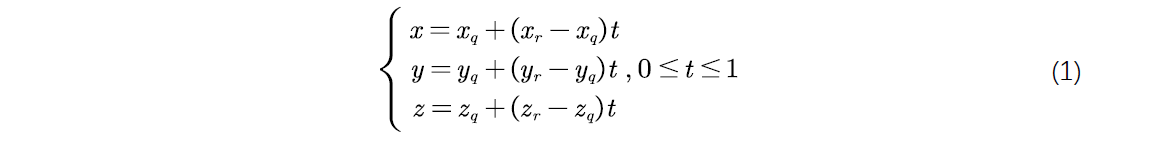然后三角形所在平面的方程用行列式表示为联立这两个方程，可以解出线段参数方程t的值，判断其是否落在[0,1]区间中，如果落在其中，那么必然有交点，且交点可以由方程(1)获得。

然后关键的是求出的交点是不是在三角形内部，这里用的是交点与三角形的三个顶点所构成的三个三角形的面积之和，是否等于整个原先的大三角形面积来确定的，即面积法。

## 2. 数值实现

作者熟练使用MATLAB软件求解问题，故而数值实现全部使用MATLAB工具。其实原理知道了，哪种语言都可以的。

### 2.1 t的符号解

直接给出符号求解的代码：

syms t xq yq zq x1 y1 z1 x2 y2 z2 x3 y3 z3 alpha beta gamma
x = xq + alpha*t;
y = yq + beta*t;
z = zq + gamma*t;
A = [x,y,z,1; x1,y1,z1,1; x2,y2,z2,1; x3,y3,z3,1];
t = solve(det(A),t)

这里我将alpha=xr-xq; beta=yr-yq; gamma=zr-zq代换了，表达式会简便一点。然后你可以得到一个老长的t关于xq、yq、zq、x1、y1、z1、x2、y2、z2、x3、y3、z3、alpha、beta、gamma的表达式：

t = -(x1*y2*z3 - x1*y3*z2 - x2*y1*z3 + x2*y3*z1 + x3*y1*z2 - ...
x3*y2*z1 - x1*y2*zq + x1*yq*z2 + x2*y1*zq - x2*yq*z1 - xq*y1*z2 +...
xq*y2*z1 + x1*y3*zq - x1*yq*z3 - x3*y1*zq + x3*yq*z1 + xq*y1*z3 - ...
xq*y3*z1 - x2*y3*zq + x2*yq*z3 + x3*y2*zq - x3*yq*z2 - xq*y2*z3 + xq*y3*z2)...
/(beta*x1*z2 - beta*x2*z1 - beta*x1*z3 + beta*x3*z1 + beta*x2*z3 -...
beta*x3*z2 - alpha*y1*z2 + alpha*y2*z1 + alpha*y1*z3 - alpha*y3*z1 -...
alpha*y2*z3 + alpha*y3*z2 - gamma*x1*y2 + gamma*x2*y1 + gamma*x1*y3 -...
gamma*x3*y1 - gamma*x2*y3 + gamma*x3*y2)

可以看出来是一个分母，一个分子，两个部分组成，因为分母不能等于0，所以要把分母先计算出来，其实分母等于0也很好理解，那就是线段跟平面平行了。

## 2.1 求交点函数

我这里就不多说了，直接贴代码

function cp = crossTriSeg(p_tri, p_seg, isPlot)
% Wan Ji, Wuhan University, 2021-9-23
% p_tri = [x1,y1,z1; x2,y2,z2; x3,y3,z3]即三角形三个顶点坐标
% p_seg = [xq,yq,zq; xr,yr,zr]即线段两个顶点坐标
% isPlot 一个逻辑判断，true则画图，否则不画图（方便显示）
cp = []; % 预设空交点
s_tri = norm(cross(p_tri(:,2)-p_tri(:,1), p_tri(:,3)-p_tri(:,1)));
if(det(s_tri)<eps)
error('输入的空间三角形三个顶点共线，请检查三个点的位置')
end
x1 = p_tri(1,1); y1 = p_tri(1,2); z1 = p_tri(1,3);
x2 = p_tri(2,1); y2 = p_tri(2,2); z2 = p_tri(2,3);
x3 = p_tri(3,1); y3 = p_tri(3,2); z3 = p_tri(3,3);
xq = p_seg(1,1); yq = p_seg(1,2); zq = p_seg(1,3);
xr = p_seg(2,1); yr = p_seg(2,2); zr = p_seg(2,3);
alpha = xr - xq;
beta = yr-yq;
gamma = zr-zq;

s =(beta*x1*z2 - beta*x2*z1 - beta*x1*z3 + beta*x3*z1 + ...
beta*x2*z3 - beta*x3*z2 - alpha*y1*z2 + alpha*y2*z1 + ...
alpha*y1*z3 - alpha*y3*z1 - alpha*y2*z3 + alpha*y3*z2 - ...
gamma*x1*y2 + gamma*x2*y1 + gamma*x1*y3 - gamma*x3*y1 - ...
gamma*x2*y3 + gamma*x3*y2);

if(abs(s)<eps)
error('线段所在直线和三角形平面平行，请检查输入！')
end

t = -(x1*y2*z3 - x1*y3*z2 - x2*y1*z3 + x2*y3*z1 + x3*y1*z2 - ...
x3*y2*z1 - x1*y2*zq + x1*yq*z2 + x2*y1*zq - x2*yq*z1 - ...
xq*y1*z2 + xq*y2*z1 + x1*y3*zq - x1*yq*z3 - x3*y1*zq + ...
x3*yq*z1 + xq*y1*z3 - xq*y3*z1 - x2*y3*zq + x2*yq*z3 + ...
x3*y2*zq - x3*yq*z2 - xq*y2*z3 + xq*y3*z2) / s;
if(t>1 || t<0)
fprintf('线段与三角形没有交点(三角形与线段外延长线有交点)')
return;
end
% 求得线段与平面的交点
x = xq + t*alpha;
y = yq + t*beta;
z = zq + t*gamma;
s1 = norm(cross([x-x1,y-y1,z-z1], [x-x2,y-y2,z-z2]));
s2 = norm(cross([x-x2,y-y2,z-z2], [x-x3,y-y3,z-z3]));
s3 = norm(cross([x-x3,y-y3,z-z3], [x-x1,y-y1,z-z1]));
if(abs(s1+s2+s3-s_tri)<1e-12)
cp = [x,y,z];
else
fprintf('线段与三角形没有交点(线段与三角形所在平面交点在三角形外面)')
end
if(isPlot)
h = patch(p_tri(:,1), p_tri(:,2), p_tri(:,3), rand(1,3));
set(h, 'facealpha',0.5,'edgecolor','k');
hold on
plot3(p_tri(:,1), p_tri(:,2), p_tri(:,3),'ro','markerfacecolor','r','markersize',8)
plot3(p_seg(:,1), p_seg(:,2), p_seg(:,3),'b-o','markerfacecolor','b','markersize',8)
if(~isempty(cp))
plot3(x, y, z,'go','markerfacecolor','g','markersize',5)
legend('三角形','三个顶点','线段','交点')
else
legend('三角形','三个顶点','线段')
end
view(54,22)
end
end

怎么用这个函数呢，其实很简单，只需要给出三角形的三个顶点坐标和线段的两个端点坐标即可，下面是调用这个函数的代码：

p_tri = [
1, 0, 0;
0, 1, 0;
0, 0, 1;
]; % 三角形三个顶点坐标

p_seg = [
0, 0, 0;
1, 1, 1;
]; % 线段两个端点坐标

cp = crossTriSeg(p_tri, p_seg, true) cp就是交点坐标，cp是空的，说明没交点;true是要画图

结果显示（这里用了format rat显示）

cp =

1/3            1/3            1/3   

可见交点坐标是(1/3,1/3,1/3)

画出的图如下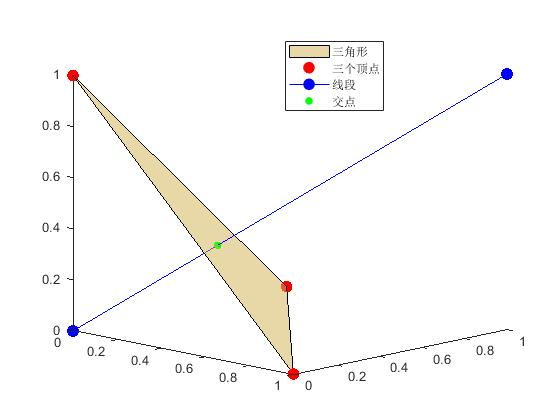可见交点求解成功！Enjoy！

## 4. 总结

本文通过线段的参数方程，以及三角形的三个顶点确定的平面方程的联合求解，根据参数t的区间判断线段和三角形的平面是否有交点，再根据面积法确定交点是否在三角形内部，得到最终的空间线段与空间三角形的坐标求解函数。

希望浏览了博主这篇文章的网友，有所收获别忘了给博主点个赞哦，有疑问可以在评论区指出呢。matlab
更多相关内容
• 为提高干涉检测的效率,提出了一种快速的三角形对相交检测算法.该算法基于三角形对的各边进行精确相交计算的原理,利用三角形边向量之间的线性相关性、线段的投影特性以及计算的中间结果,提高了三角形对相交检测的效率...
• ​我们视线所及之处的空间似乎在朝着四面八方无限延伸，这不禁让人遐想：宇宙究竟是什么形状的？目前，对宇宙的形状有三种主要的假设：宇宙是平坦的，像纸张一样；宇宙的曲率为正，像球体一样；宇宙的曲率为负，像...

​我们视线所及之处的空间似乎在朝着四面八方无限延伸，这不禁让人遐想：宇宙究竟是什么形状的？

目前，对宇宙的形状有三种主要的假设：

1. 宇宙是平坦的，像纸张一样；
2. 宇宙的曲率为，像球体一样；
3. 宇宙的曲率为，像马鞍一样。

因此要探讨宇宙的形状，我们必须了解这三种不同的空间几何。平面几何学关于平面几何学的故事，我们需要追溯到很久很久以前，当欧几里得首次将我们生活的世界的几何原理公式化的时候。他在著作《几何原本》中提出了5个几何公设：

1. 任意两点可以通过一直线连接；
2. 任意线段都能延伸成一直线；
3. 任意线段可以一个端点为圆心该线段为半径作圆；
4. 所有直角都全等；
5. 若一条直线与两条直线相交，使同侧的两角之和小于两个直角，那么这两条直线无限延伸必定相交。

19世纪，勒让德证明了第5公设等价于“三角形内角之和等于两个直角。”这一公设下的几何也被称为“欧几里得几何”或“平面几何”。在这种几何中，两条平行的直线永远不会相交，三角形的内角和总是180度。与这种几何相对应的是曲率为0的平坦宇宙。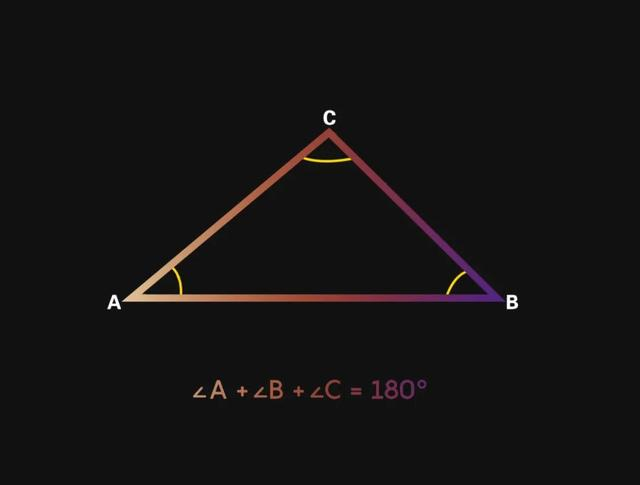与前4条公设相比，第5条公设更加复杂。欧几里得自己也隐约觉得，第5公设好像不似其他几条那般完美。直到19世纪，数学家才终于找到了一个让第5公设不成立的几何学例子，证明了这个在2000多年的时间里一直被视为正确的公设，的确不完美。

这一发现也直接导致了非欧几里得几何的诞生。而这一发现也惊喜地促成了广义相对论的诞生，彻底地颠覆了我们的宇宙观。在非欧几里得几何空间中，无论宇宙是正向弯曲还是负向弯曲，事物都开始变得奇怪起来。球面几何学当宇宙的曲率为正时，两条平行的曲线会向一个单点倾斜，与之对应的是球面几何，在这种几何中，欧几里得的第5公设失效了。

在这种几何中，平面几何中的“直线”变成了一个大圆，也就是由过球心的平面与球面的交线构成的圆。球面上的三角形内角和也不再等于180度，而是稍大于180度。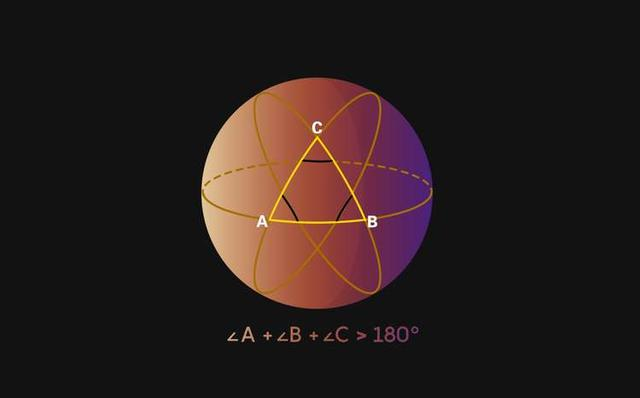或许对于球面上的那些非常小的三角形来说，我们很难察觉到这一点。这是因为以一个非常小的三角形的视角来看，球面几乎是平坦的。这也是为何对于生活在地球这样一个球面上的我们来说，却用了如此长的时间才从平面几何思维转换到球面几何。很显然，当讨论的球面上的大三角形时，就能明显察觉其内角大于180度。双曲几何学当宇宙的曲率为负时，意味着两条平行的直线会永远发散，与之对应的是双曲线几何。在双曲线几何中，欧几里得的第5公设以和球面几何类似却又恰好反向的理由失效了，不过前4个公设在双曲几何中仍然成立。

与球面几何相比，双曲线几何更难以被可视化。不同于球面几何的向内闭合，双曲几何是向外张开的。一种可被用来展示双曲几何的方法叫做庞加莱半平面模型(Poincaré half-plane model)。这个模型与“真实”的双曲空间之间的关系，有点类似于平面地图与球面世界之间的关系。举个例子，驾驶一架飞机从北京直飞伦敦，那么在平面地图上画出的路线不会是直的，而是弯曲的。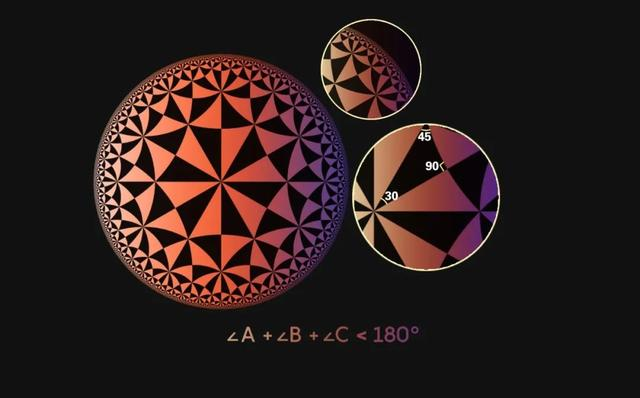在欧几里得几何中，圆的周长与半径成正比；但在双曲几何中，圆周长与半径成指数关系。如果放大双曲圆盘的边界，就会看到在那里堆积着大量的三角形。在双曲几何中，三角形的内角是小于180度的。以庞加莱圆盘中的三角形为例，其内角和为165度。宇宙的形状

了解不同几何的性质，对于思考宇宙的大尺度形状至关重要。要了解宇宙的形状，研究人员需要测量宇宙中物质的密度。因为根据爱因斯坦的广义相对论，空间本身是可以被质量弯曲的。因此，通过比较宇宙的临界密度与实际密度，计算出宇宙的空间曲率，从而推断宇宙是“开放的”、“闭合的”，还是“平坦的”。

如果宇宙的实际密度大于临界密度，它就包含足够多的质量来最终阻止膨胀，那么这就是一个闭合的宇宙，有一个球形的形状。如果宇宙的实际密度小于临界密度，就意味着宇宙中没有足够的物质来阻止宇宙的膨胀，宇宙会永远膨胀下去，这就是所谓的开放宇宙，其形状会像马鞍的表面一样弯曲。但如果宇宙恰好包含了足够的质量使膨胀停止，它的实际密度将等于临界密度，这种情况下，宇宙被认为是平坦的。

这些问题的答案都“刻写”在天空中，隐藏在从四面八方朝我们袭来的宇宙微波背景(CMB)辐射里。根据目前的CMB证据表明，平面几何最有可能是正确的：研究人员测得，宇宙的曲率为0，这意味着可观测宇宙基本上是平滑且均匀的，即空间的局部结构在每个点和每个方向上看起来都一样。

尽管如，在更大的尺度上，宇宙仍有可能是弯曲的，只是这超出了我们的感知范围。就像站在大平上的我们可能会觉得地球是平的。而目前我们所知道的，只是可观测的宇宙几乎是平坦的。

研究宇宙的形状其实是在为研究宇宙的起源提供线索，它同时也是我们推测宇宙的最终命运的关键信息。它与宇宙中物质的形状和密度，以及暗能量的强度息息相关，而这一切都将最终决定宇宙是会在大挤压中收缩回去，还是在热寂寞中四散死亡。

展开全文• 这次我们计算依稀空间三角形内心（内切圆的圆心）。 问：已知世界空间中任意三角形和顶点坐标，求其内心坐标和法向量。如下： 一般平面三角形内心求法：任意两角的平分线相交点即为内心，如图： 这也好...

之前计算过外心了，这次我们计算一下空间三角形内心（内切圆的圆心）。

问：已知世界空间中任意三角形和顶点坐标，求其内心坐标。如下：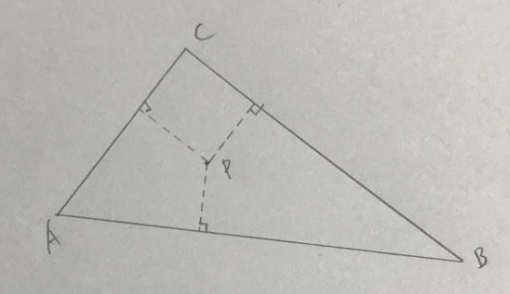一般平面三角形内心求法：任意两角的平分线相交点即为内心，如图：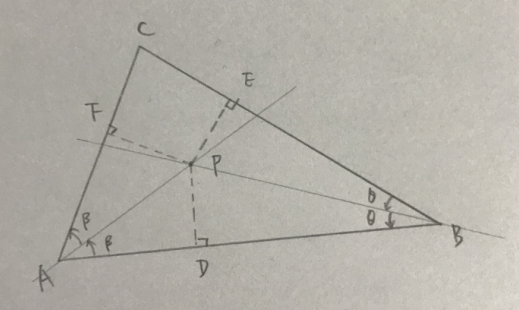这也好理解：△ABC任意两角角度平分后交于P点，做垂线PD、PE、PF，可以组成四个直角三角形，则垂线PD、PE、PF的长度相同，所以交点P即为内心。

那么求空间三角形内心的关键：求空间角平分线，例如求∠BAC平分线AP，如下：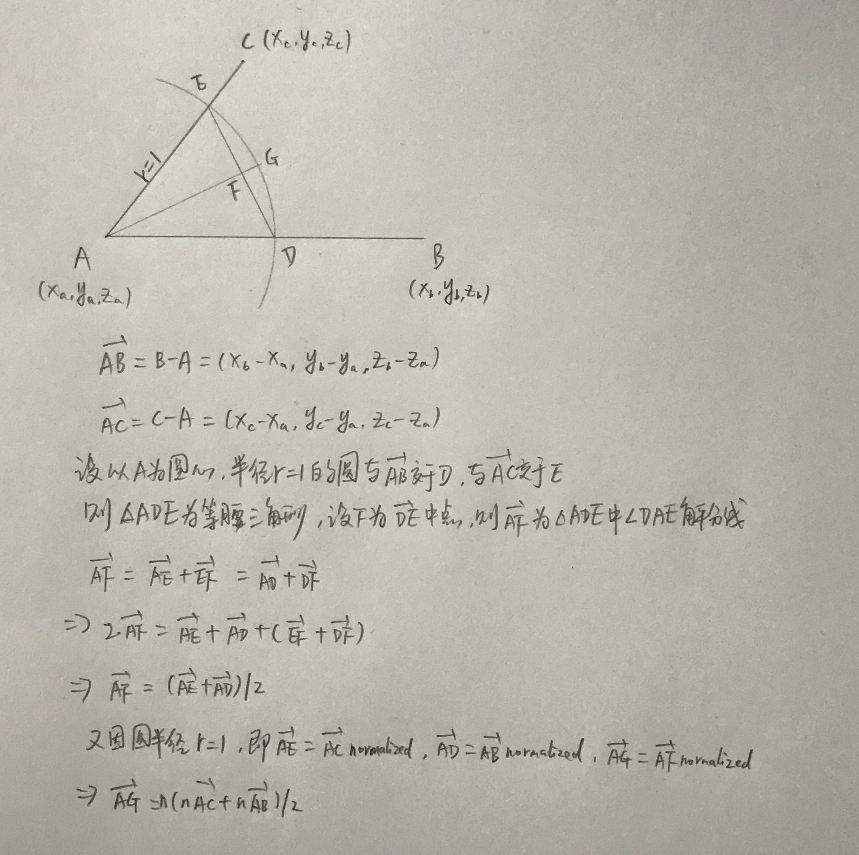我们得到单位平分线AG的表达式。

接下来就是求△ABC平分线的交点P(x,y,z)，也就是内心：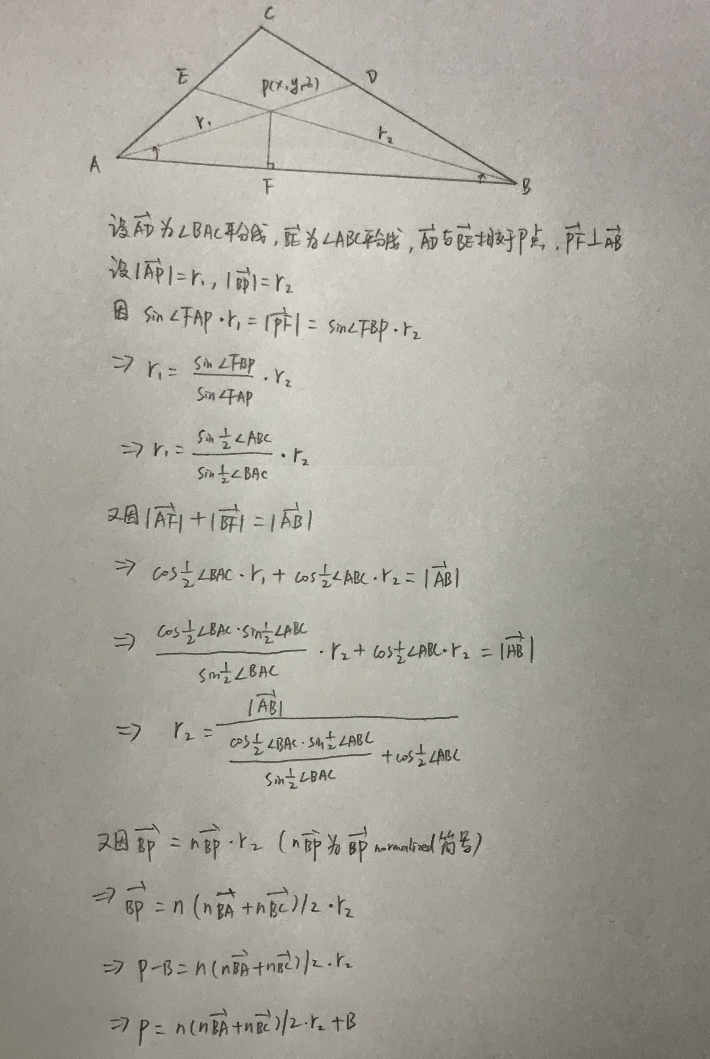主要是通过直角三角形性质，求得r2或者r1的长度，即可得到P点的坐标表达式。其中∠ABC和∠BAC的求法如下：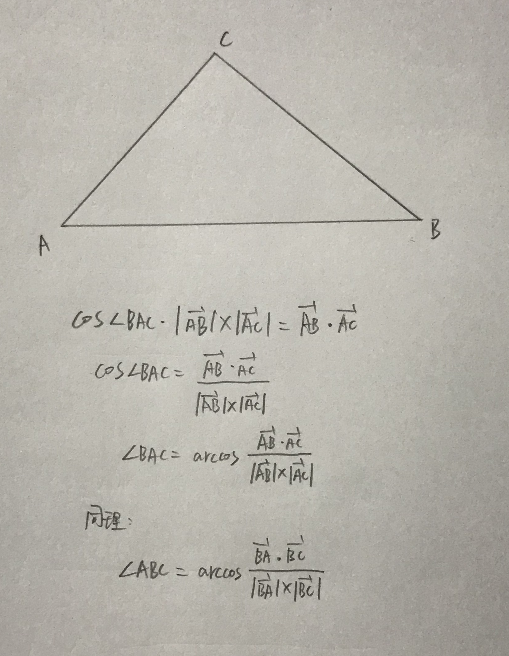主要就是通过点积求得角度。

接下来就是通过代码来具体实现一下，看下我们推导是否正确：

using UnityEngine;

public class TriangleInsideCenter : MonoBehaviour
{
public Transform TA;
public Transform TB;
public Transform TC;
public Transform TP;

void Start()
{
float deg = 30f;
float sin = Mathf.Sin(deg * Mathf.Deg2Rad);
float cos = Mathf.Cos(deg * Mathf.Deg2Rad);

float arcsin = Mathf.Asin(sin) * Mathf.Rad2Deg;
float arccos = Mathf.Acos(cos) * Mathf.Rad2Deg;
}

void Update()
{
Vector3 p = CalculateInsideCenter(TA.position, TB.position, TC.position);
TP.position = p;
#if UNITY_EDITOR
Debug.DrawLine(TA.position, TB.position, Color.black);
Debug.DrawLine(TB.position, TC.position, Color.black);
Debug.DrawLine(TC.position, TA.position, Color.black);

Debug.DrawLine(TA.position, p, Color.white);
Debug.DrawLine(TB.position, p, Color.white);
Debug.DrawLine(TC.position, p, Color.white);
#endif
}

private Vector3 CalculateInsideCenter(Vector3 A, Vector3 B, Vector3 C)
{
Vector3 AB = B - A;
Vector3 AC = C - A;
Vector3 BA = A - B;
Vector3 BC = C - B;

Vector3 nBA = BA.normalized;
Vector3 nBC = BC.normalized;

float radBAC = Mathf.Acos(Vector3.Dot(AB, AC) / (AB.magnitude * AC.magnitude));
float radABC = Mathf.Acos(Vector3.Dot(BA, BC) / (BA.magnitude * BC.magnitude));

Vector3 P = ((nBA + nBC) / 2f).normalized * r2 + B;
return P;
}
}


效果如图：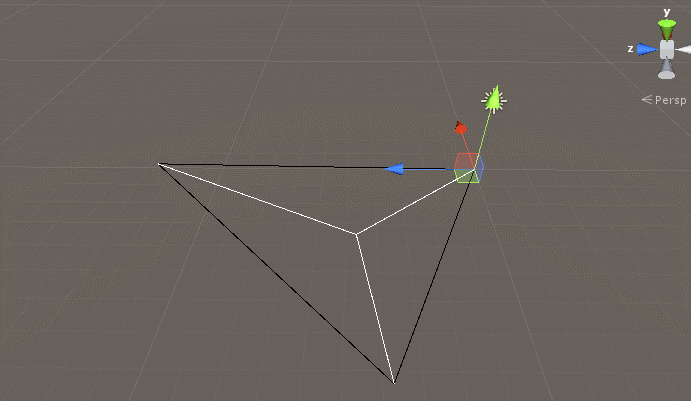估计这种看不出来结果是否正确，我们得将内心P到△ABC每条边的垂点和模长计算出来才行，如下：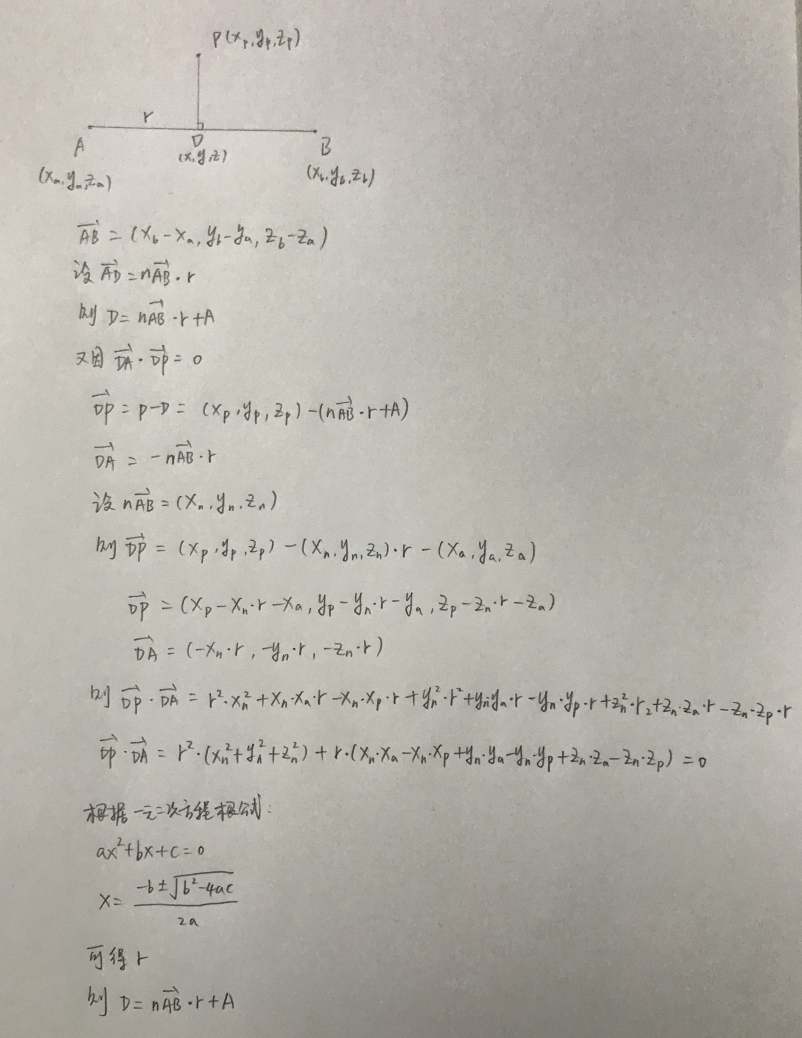可以根据向量表达式和点积以及一元二次方程求根得到垂点D的表达式，接下来通过代码实现如下：

using UnityEngine;

public class TriangleInsideCenter : MonoBehaviour
{
public Transform TA;
public Transform TB;
public Transform TC;
public Transform TP;

void Start()
{
float deg = 30f;
float sin = Mathf.Sin(deg * Mathf.Deg2Rad);
float cos = Mathf.Cos(deg * Mathf.Deg2Rad);

float arcsin = Mathf.Asin(sin) * Mathf.Rad2Deg;
float arccos = Mathf.Acos(cos) * Mathf.Rad2Deg;
}

void Update()
{
Vector3 A = TA.position;
Vector3 B = TB.position;
Vector3 C = TC.position;
Vector3 P = CalculateInsideCenter(TA.position, TB.position, TC.position);
TP.position = P;
#if UNITY_EDITOR
Debug.DrawLine(A, B, Color.black);
Debug.DrawLine(B, C, Color.black);
Debug.DrawLine(C, A, Color.black);

Vector3 D = CalculateVerticalVertex(A, B, P);
Vector3 E = CalculateVerticalVertex(B, C, P);
Vector3 F = CalculateVerticalVertex(C, A, P);

Debug.DrawLine(D, P, Color.white);
Debug.DrawLine(E, P, Color.white);
Debug.DrawLine(F, P, Color.white);

Debug.LogFormat("DP = {0} EP = {1} FP = {2}", Vector3.Distance(D, P), Vector3.Distance(E, P), Vector3.Distance(F, P));
#endif
}

private Vector3 CalculateInsideCenter(Vector3 A, Vector3 B, Vector3 C)
{
Vector3 AB = B - A;
Vector3 AC = C - A;
Vector3 BA = A - B;
Vector3 BC = C - B;

Vector3 nBA = BA.normalized;
Vector3 nBC = BC.normalized;

float radBAC = Mathf.Acos(Vector3.Dot(AB, AC) / (AB.magnitude * AC.magnitude));
float radABC = Mathf.Acos(Vector3.Dot(BA, BC) / (BA.magnitude * BC.magnitude));

Vector3 P = ((nBA + nBC) / 2f).normalized * r2 + B;
return P;
}

private Vector3 CalculateVerticalVertex(Vector3 A, Vector3 B, Vector3 P)
{
Vector3 AB = B - A;
Vector3 nAB = AB.normalized;

float Xn = nAB.x;
float Yn = nAB.y;
float Zn = nAB.z;

float Xa = A.x;
float Ya = A.y;
float Za = A.z;

float Xp = P.x;
float Yp = P.y;
float Zp = P.z;

float a = Xn * Xn + Yn * Yn + Zn * Zn;
float b = Xn * Xa - Xn * Xp + Yn * Ya - Yn * Yp + Zn * Za - Zn * Zp;
float c = 0;

float[] rs = CalculateQuadraticEquation(a, b, c);
Vector3 D = Vector3.zero;
//剔除模长r为0的情况
if (rs != 0)
{
D = nAB * rs + A;
}
else if (rs != 0)
{
D = nAB * rs + A;
}
return D;
}

private float[] CalculateQuadraticEquation(float a, float b, float c)
{
float x1 = (-b + Mathf.Sqrt(b * b - 4 * a * c)) / (2 * a);
float x2 = (-b - Mathf.Sqrt(b * b - 4 * a * c)) / (2 * a);
return new float[]
{
x1,
x2
};
}
}


最后效果如下：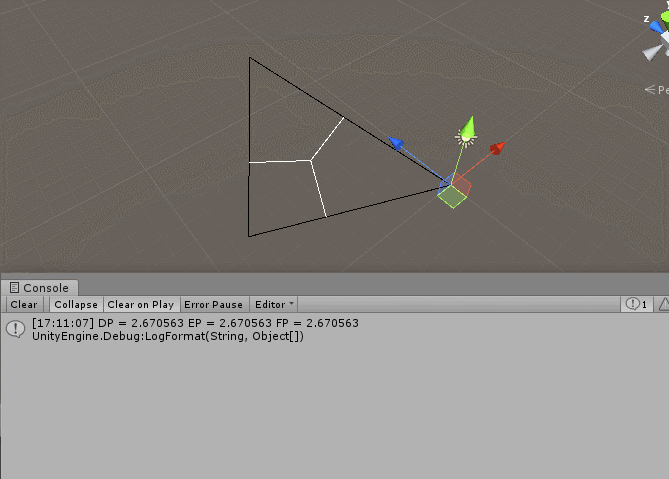可以通过图形和打印看得出来结果正确。

展开全文• 这次来计算一下任意空间三角形的外心（也就是外接圆的圆心）。

这次来计算一下世界空间三角形的外心（也就是外接圆的圆心）和外心为起点的平面法向量。

问题：假设我们世界空间中有一个任意三角形，且已知三角形各个顶点ABC的坐标，求三角形的外心P和外心所在的法向量n，如下图：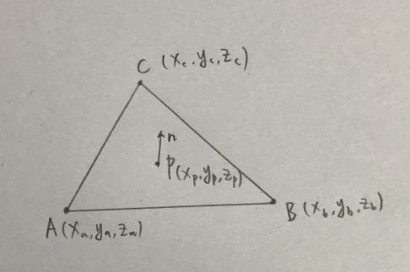如果依稀还记得初中几何，就知道一个平面三角形外心计算规则：三角形任意两边的中垂线交点，则为外心。三角形所在平面法向量：以一顶点为端点的逆时针方向两条边的叉积则为平面法向量。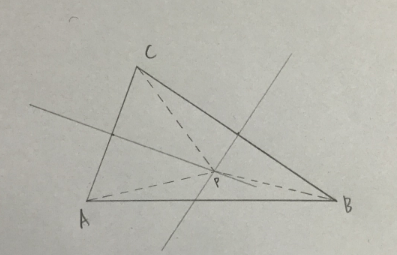其实一眼就看得出来原理：两条中垂线组成的三角形APC、BPC为等腰三角形，则AP=BP=CP，即P点位外心。

那么我们任意选取三角形一边如AC，计算中垂线DP，根据AP=BP，则可以求出外心P的坐标，如下：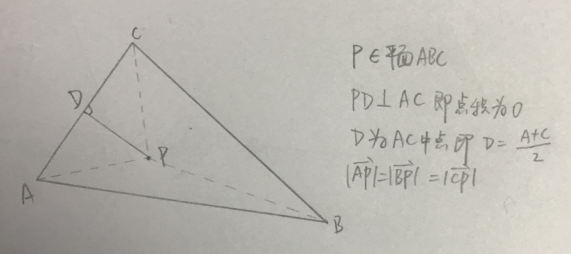假设△ABC的外心为P(x,y,z)，条件整理如下：

1.△ABC各个顶点坐标已知

2.P(x,y,z)在△ABC所处的平面中，可以带入平面方程计算（平面方程理解

3.PD⊥AC，即dot(PD,AC) = 0

4.D = (A+C)/2

5.AP、BP、CP模长相同

将这些条件用来计算P(x,y,z)，我们尝试一下：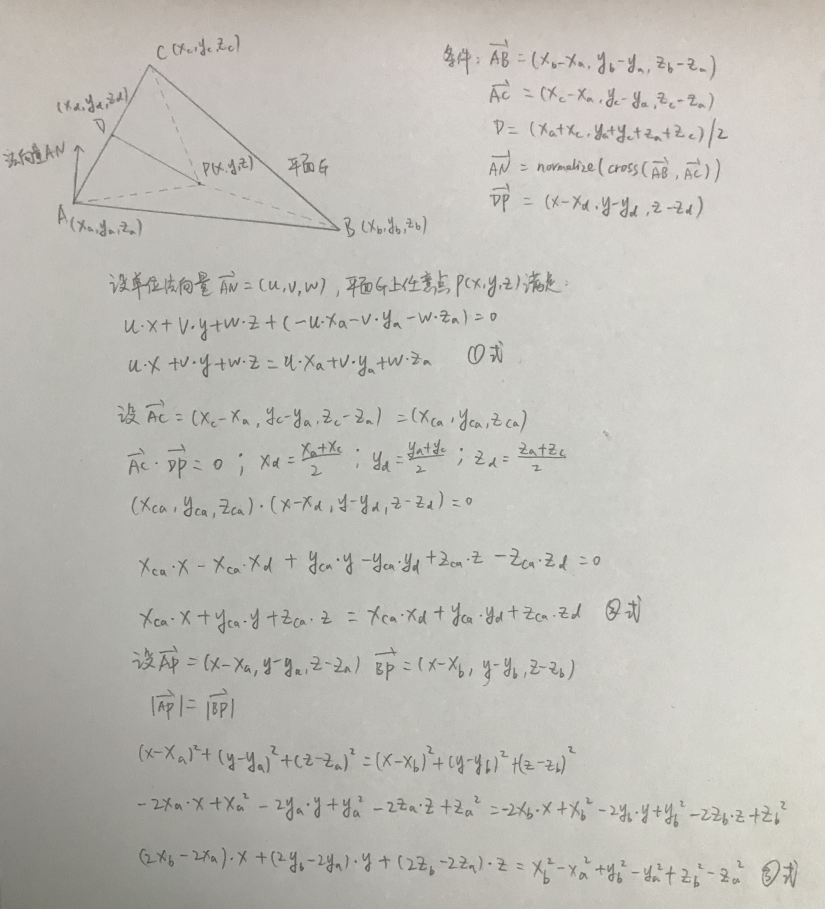我们通过条件整理出①②③三个三元一次方程，接下来我们通过三元一次方程求解，如下：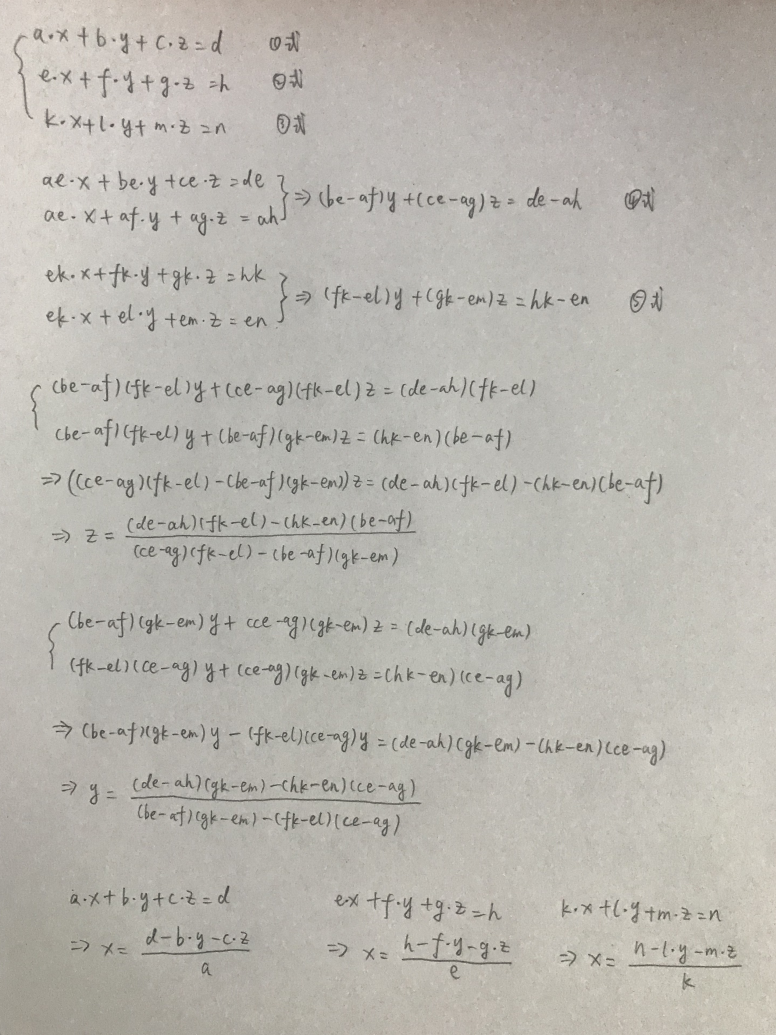将①②③带入三元一次方程解：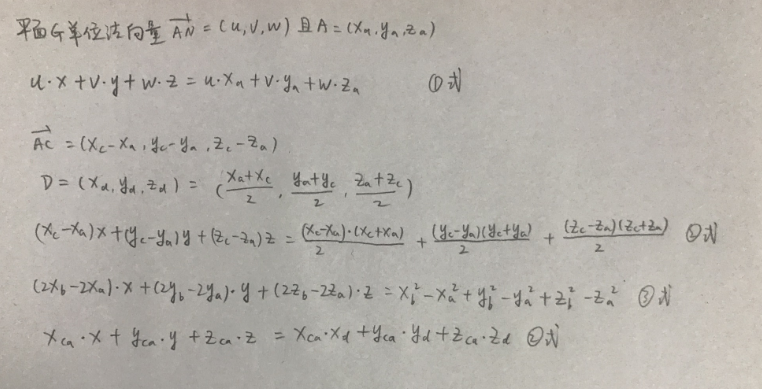方程的参数和根巨复杂，我自己推导起来都要不停的返回瞄一下是否参数写错了，小伙伴们可能需要很仔细的查看推导过程。

当然我们还是要用c#代码来实现一下：

using System.Collections;
using System.Collections.Generic;
using UnityEngine;

public class TriangleVerticeCenter : MonoBehaviour
{
public Transform TA;
public Transform TB;
public Transform TC;
public Transform TP;

void Start()
{

}

void Update()
{
Vector3 p = CalculateTriangleOutCircleCenter(TA.position, TB.position, TC.position);
TP.position = p;
//PN法向量
Vector3 PB = TB.position - p;
Vector3 PC = TC.position - p;
Vector3 PN = Vector3.Cross(PB, PC).normalized;
Vector3 n = p + PN;
#if UNITY_EDITOR
Debug.DrawLine(TA.position, TB.position, Color.black);
Debug.DrawLine(TB.position, TC.position, Color.black);
Debug.DrawLine(TC.position, TA.position, Color.black);

Debug.DrawLine(TA.position, p, Color.white);
Debug.DrawLine(TB.position, p, Color.white);
Debug.DrawLine(TC.position, p, Color.white);

Debug.DrawLine(p, n, Color.red);

Debug.LogFormat("AP = {0} BP = {1} CP = {2}", Vector3.Distance(TA.position, p), Vector3.Distance(TB.position, p), Vector3.Distance(TC.position, p));
#endif
}

private Vector3 CalculateTriangleOutCircleCenter(Vector3 A, Vector3 B, Vector3 C)
{
float Xa = A.x;
float Ya = A.y;
float Za = A.z;

float Xb = B.x;
float Yb = B.y;
float Zb = B.z;

float Xc = C.x;
float Yc = C.y;
float Zc = C.z;

Vector3 D = (A + C) / 2;
float Xd = D.x;
float Yd = D.y;
float Zd = D.z;

//单位法向量AN
Vector3 AB = B - A;
Vector3 AC = C - A;
Vector3 AN = Vector3.Cross(AB, AC).normalized;

float u = AN.x;
float v = AN.y;
float w = AN.z;

//构建三元一次方程参数
float a = u;
float b = v;
float c = w;
float d = u * Xa + v * Ya + w * Za;

float e = Xc - Xa;
float f = Yc - Ya;
float g = Zc - Za;
float h = (Xc - Xa) * (Xc + Xa) / 2 + (Yc - Ya) * (Yc + Ya) / 2 + (Zc - Za) * (Zc + Za) / 2;

float k = 2 * Xb - 2 * Xa;
float l = 2 * Yb - 2 * Ya;
float m = 2 * Zb - 2 * Za;
float n = Xb * Xb - Xa * Xa + Yb * Yb - Ya * Ya + Zb * Zb - Za * Za;

float[] equa = CalculateTernaryEquation(a, b, c, d, e, f, g, h, k, l, m, n);
Vector3 P = new Vector3(equa, equa, equa);
return P;
}

private float[] CalculateTernaryEquation(float a, float b, float c, float d, float e, float f, float g, float h, float k, float l, float m, float n)
{
float z = ((d * e - a * h) * (f * k - e * l) - (h * k - e * n) * (b * e - a * f)) / ((c * e - a * g) * (f * k - e * l) - (b * e - a * f) * (g * k - e * m));
float y = ((d * e - a * h) * (g * k - e * m) - (h * k - e * n) * (c * e - a * g)) / ((b * e - a * f) * (g * k - e * m) - (f * k - e * l) * (c * e - a * g));
float x = 0;
if (a != 0)
x = (d - b * y - c * z) / a;
else if (e != 0)
x = (h - f * y - g * z) / e;
else if (k != 0)
x = (n - l * y - m * z) / k;
return new float[] { x, y, z };
}
}


效果如下：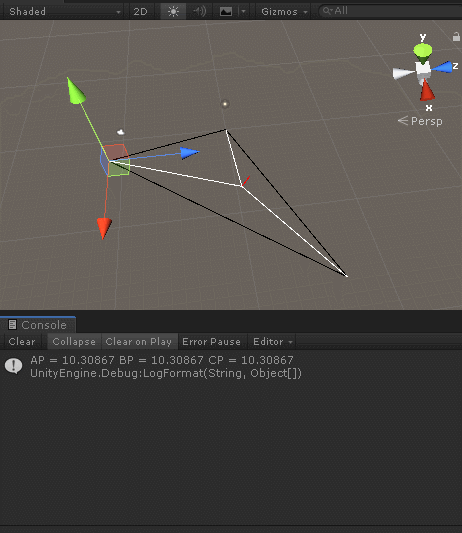可以通过图形标注和打印信息看得出来，外接圆心和法向量计算正确。

展开全文• 空间三角形不能直接说是顺时针还是逆时针，必须是从某个方向看下去来进行判定，比如往z轴负方向看，该空间三角形是顺时针，但往z轴正方向看该空间三角形又是逆时针。那么，到底应该采用什么样的方法来判定一个空间...
• Devillers & Guigue算法( 简称Devillers 算法) 通过三角形各顶点构成的行列式正负的几何意义来判断三角形中点、线、面之间的相对位置关系,从而判断两三角形是否相交。快速 相交 c++
• 接之前最简理解空间射线与平面交点的场景。 获得射线打在三角形上的交点（之后称为“打点”）后，假设三角形为p1,p2,p3，打点为c，想求直线p1c和p2p3的交点。 转化为射线与p2p3的法平面交点，于是可以套用同一公式...物理引擎 渲染引擎
• HTML5 Canvas科技感三角形二维动画特效，蓝色科技感十足的网页背景动画效果。
• “中心”：如果给定顶点的分量，则计算三角形的重心、内心、外心和正交心，并绘制三角形和选定的中心。 写： >> 帮助中心用途matlab
• 检测空间三角形相交 C#,通过计算行列式的值来判断空间内三角形点的分布情况，再计算是否相交，快速排除不相交的情况。
•计算几何 算法
• 参考文献： 基于三角形法向量的离散点三维构网方法
• 我们将三角形放置在二维平面坐标系中， 并设其三点坐标为，，，如下图所示：我们采用构造已知图形面积的方法来求解未知图形面积的方法，所以我们在坐标系里对三角形ABC三个顶点做x轴和y轴的垂线，于是三角形ABC的...
• 讲述了空间射线与三角形相交的常规算法和优化算法，包括具体的理论推导与C++实现。相交 算法 C++
• 请查看右侧的示例选项卡 (doc) 以获取完整说明。 下载后，在 Matlab 控制台中键入“doc sample_triangle”或“help sample_triangle”以获得支持。matlab
• 已知二维空间三角形三个顶点坐标为：（x1, y1 )，（x2,y2 )，( x3, y3） 三角形面积公式： 公式(1)S = abs(x1*(y2-y3) + x2*(y3-y1) + x3*(y1-y2))/2; 公式(2)-海伦公式： &...
• 射线和三角形的相交检测（ray triangle intersection test）
• 在几何相关的数学建模问题中，会遇到已知三角形顶点空间坐标，判断空间任意点是否在三角形内部的情况，本文给出了一种判断空间点是否在三角形内的简单算法，并通过Matlab进行实现，同时支持矩阵运算。 算法基本...matlab 算法
• //满足右手定则（按夹角为锐角，顺时针），顺时针由a到b， //大拇指的方向就是e的方向，AP x AB 与 AC x AB 叉乘的结果是同向的， //方向都是垂直三角形ABC这个平面朝上的， Vector3 v1 = AB.Cross(AC) ;...
• CSS3 SVG三角形不断放大特效是一款css3 animation基于svg绘制旋转放大的透明三角形动画特效。CSS3 SVG
• 为此，该演示使用基于轴对齐的边界框的四叉树数据结构来加快对网格中三角形的搜索。 根据搜索点，检索四叉树区域的所有三角形，并显示有关搜索的相关信息。 可以通过三个参数对四叉树的创建进行参数设置：-最大树...开源软件
• 平面中判断一个点是否在三角形内部有很多方法，其中一个比较快的方法是：利用叉乘计算PA，PB，PC，是否同向。代码如下： def Triangle(PointA,PointB,PointC,P): # PointA，PointB，PointC为三角形的三个顶点，P为...python
• 转自：http://hi.baidu.com/chuckhao/blog/category/%BC%C6%CB%E3%BB%FA%CD%BC%D0%CE%D1%A7空间三角形与射线相交并求交点的重心坐标系表示2008-05-05 13:41 在光线追踪中一个重要的步骤就是从屏幕上投射出一道光线...图形 算法
• 我们知道三角形的面积=1/2×a×b×sinC，其中C为ab两边的夹角。 运用叉乘公式（matlab里用cross()函数实现），我们可以得到absinC，但这时候结果还是一个三维向量，用norm()函数取向量的模长即可。 求三角形面积函数...
• 那么在空间以AB为斜边构造垂直于H面的直角三角形,其中平行于H面的直角边长为ab,而垂直于H面的直角边长为a’b’的上下距离. 如图. 将此三角形翻转为与H面平行,平移至与H面重合,再让原本平行于H面的直角边于ab重合,...
• 最近在看recast&amp;detour源码的时候有遇到许多数学上的...// 三角形空间点 a b c // 输出参数 t static bool intersectSegmentTriangle(const float* sp, const float* sq, const float* a, con......